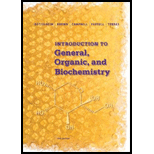# 5-55 Answer true or false. (a) Partial pressure is the pressure that a gas in a container would exert if it were alone in the container. (b) The units of partial pressure are grams per liter. (c) Dalton's law of partial pressures states that the total pressure of a mixture of gases is the sum of the partial pressures of each gas. (d) If 1 mole of gas at STP is added to 22.4 L of N 2 at STP, the final pressure in the 22.4 L container will be 1.00 atm.### Introduction to General, Organic a...

11th Edition
Frederick A. Bettelheim + 4 others
Publisher: Cengage Learning
ISBN: 9781285869759### Introduction to General, Organic a...

11th Edition
Frederick A. Bettelheim + 4 others
Publisher: Cengage Learning
ISBN: 9781285869759

#### Solutions

Chapter 5, Problem 5.55P
Textbook Problem

## Expert Solution

### Want to see the full answer?

Check out a sample textbook solution.

### Want to see this answer and more?

Experts are waiting 24/7 to provide step-by-step solutions in as fast as 30 minutes!*

*Response times vary by subject and question complexity. Median response time is 34 minutes and may be longer for new subjects.